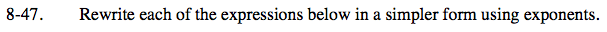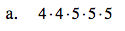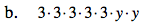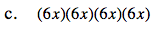Home > ACC7 > Chapter cc38 > Lesson cc38.2.1 > Problem8-47

8-47.
1. Rewrite each of the expressions below in a simpler form using exponents. Homework Help ✎

1. 4 · 4 · 5 · 5 · 5

2. 3 · 3 · 3 · 3 · 3 · y · y

3. (6x)(6x)(6x)(6x)Notice how many 4's there are and how many 5's there are. The exponent refers to how many times a number is multiplied by itself.

Since there are two 4's and three 5's:

42 · 53Use the same method as in (a).Use the same method as in (a).

(6x)4